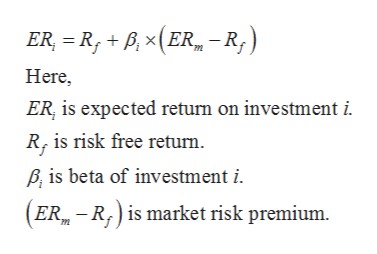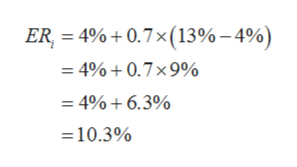# Based on current dividend yields and expected capital gains, the expected rates or return on portfolios A and B are 12% and 18%, respectively.  The beta of A is 0.7 while that of B is 1.6.  The T-bill rate is currently 4% while the expected rate of return of the S&P500 Index is 13%.  The standard deviation of portfolio A is 14% annually, while that of B is 26%, and that of the index is 15%.If you currently hold a market-index portfolio, would you chose to add either of these portfolios to your holdings?

Question
160 views

Based on current dividend yields and expected capital gains, the expected rates or return on portfolios A and B are 12% and 18%, respectively.  The beta of A is 0.7 while that of B is 1.6.  The T-bill rate is currently 4% while the expected rate of return of the S&P500 Index is 13%.  The standard deviation of portfolio A is 14% annually, while that of B is 26%, and that of the index is 15%.

If you currently hold a market-index portfolio, would you chose to add either of these portfolios to your holdings?

check_circle

star
star
star
star
star
1 Rating
Step 1

Capital asset pricing model can be used to study the how systematic risk on a particular asset is linked to expected return on the same asset.

The formula to calculate expected return using CAPM model is given below:help_outlineImage TranscriptioncloseER = R,+ P,(ER-R,) Here ER, is expected return on investment i. R, is risk free returm P, is beta of investment i. (ER-R) is market risk premium. fullscreen
Step 2

To calculate expected return on portfolio A,

Substitute 4% for Rf, 0.7 for βi, 13% for ERm in the above formula,help_outlineImage Transcriptionclose4%+0.7x (13% - 4%) ER = 4% + 0.7 x 9% = 4%+6.3% 10.3 % fullscreen
Step 3

To calculate expected return on portfolio B,

Substitute 4% for Rf, 1.6 for βi , 13...

### Want to see the full answer?

See Solution

#### Want to see this answer and more?

Solutions are written by subject experts who are available 24/7. Questions are typically answered within 1 hour.*

See Solution
*Response times may vary by subject and question.
Tagged in